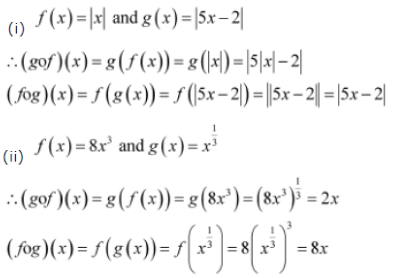# Find gof and fog, if (i) f(x) = |x| and g(x) = 15x - 21

Find gof and fog, if
(i) f(x) = |x| and g(x) = 15x - 21
(ii) f(x) = 8x3 and g(x) = x 1/3